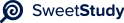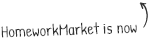•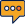0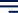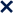1 / 1100%Some statements are more dicult to prove using a direct
proof so instead we use an indirect proof. An indirect proof
may include a proof of contradiction or a proof of
contrapositive. A proof of contradiction starts with the
assumption that the theorem is false, which eventually
contrapositive, on the other hand, for a conditional
statement,
a→b
, we assume the conclusion is false, therefore the hypothesis is false,
¬b→¬a
. A simple example of proof of contrapositive is:
Theorem: If#5n+3#is an odd number, then n must be an
even number.
Proof:
Assume n represents an odd integer (the negation of the
conclusion).
Since n is an odd integer,#n=2k+1#for some integer k.
We then substitute n into the equation to
get#5(2k+1)+3.
10k+8=2(5k+4)
(5k+4)#is still an integer.
When you multiply any integer by 2, it results in an even
integer (the negation of the hypothesis).